# Pixel Binning Methods

## Introduction

This vignette is intended to explain the implications of different binning methods for doing color similarity analyses with the colordistance package.

colordistance comes with two binning functions: getImageHist() and getKMeanColors() (or getHistList() and getKMeansList() for multiple images at once), which categorize colors in a picture using two popular approaches for pixel clustering. Depending on the dataset, the method you choose can have a dramatic impact on your final results. The following explanations should hopefully clarify the differences, and which binning method is most appropriate for a certain dataset.

## Why binning?

Binning is a way of grouping continuous data into categories defined by specific ranges – shoe sizes are a good example of binning (there are certainly more unique foot dimensions than commercially available shoe sizes). In image processing, the first step in measuring color composition of an image is usually to bin all the pixels into a color histogram. This obviously comes at the cost of reducing the variation in the image, but it has a couple of major advantages for image comparisons:

1. It reduces and normalizes the amount of data we need to compare across images.

If instead of binning, we compared every pixel in an image to a pixel in another image, we would have three major computational problems:

• We would have to decide which pixels to compare to which other pixels;
• Even a simple paired comparison would require thousands or millions of calculations per pair of images;
• And the images almost certainly won’t have the same number of pixels.

By binning, we can compare apples to apples by comparing bins with the same boundaries from different images. And when we do that, we’re only comparing a finite number bins in one image to the exact same number of bins in another image, which is much quicker than trying to do it for every pixel, especially when much of the pixel-level variation isn’t important for the analysis.

2. It allows us to measure the amount of every color in the image.

For example, take the following picture of a Heliconius butterfly (Meyer (2006)):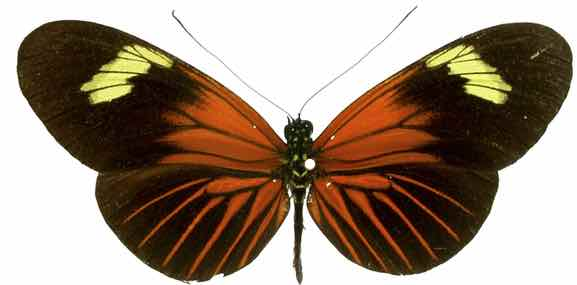We could very reasonably say that there are only 3 real colors on this butterfly: black, red, and yellow. But when we plot the individual pixels in the actual image…

# Note: any valid filepath will work; this line is specific to
# example data that comes with the package
Heliconius_08 <- system.file("extdata", "Heliconius/Heliconius_B/Heliconius_08.jpeg", package="colordistance")
colordistance::plotPixels(Heliconius_08, lower = rep(0.8, 3), upper = rep(1, 3))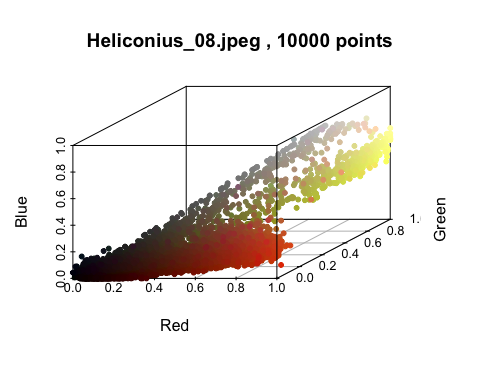(See the introduction for more information on the plotPixels() function.)

As you might expect, although we can see black, red, and yellow pixels, they’re not all centered in a single place because of noise, lighting variation, and of course actual minor color differences in the animal itself when it was photographed. But if we define a certain range for black pixels in RGB color space, we can just count the proportion of pixels in that range:

binnedButterfly <- colordistance::getImageHist(Heliconius_08, bins = 2, lower = rep(0.8, 3), upper = rep(1, 3), plotting = TRUE)
## RGB and HSV are device-dependent, perceptually non-uniform color spaces. See 'Color spaces' vignette for more information.
##
## Using 2^3 = 8 total bins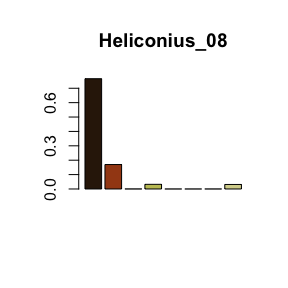(For more information in getImageHist(), see the ‘Histogram method’ section.)

And we can confidently say that 75% of the pixels in the butterfly were in the “black” range we defined. We’re treating all pixels in that bin as if they’re the same color, but in this case we can say that the simplification doesn’t sacrifice important information. It’s pretty accurate to say that the majority of the butterfly is black; that some of the pixels in the black regions are dark brown or dark grey is not meaningful biological variation for this image. Of course, there are situations where those more subtle color variations do matter, in which case you’d need smaller bins to break those pixels up into tighter ranges.

## Histogram method

Both the getImageHist() and getHistList() functions in colordistance use color histograms to bin the pixels in an image.

Each color channel – either red, green and blue (if using RGB) or hue, saturation, and value (if using HSV) – is divided into ranges of equal size. Each combination of ranges from the three channels forms a 3D “bin”. For example, if we chose to divide the blue channel into 2 bins, our ranges in the blue channel would be $$0 \leq B \leq 0.5$$ and $$0.5 < B \leq 1$$1.

If we did this for each channel, we’d have a total of $$2^{3}=8$$ bins. A pixel with RGB coordinates of [0.2, 0.8, 0.1] would be in the bin defined by RGB bounds of [0, 0.5], [0.5, 1], and [0, 0.5] – a pixel with the value [0.4, 0.6, 0.4] would be in that same bin. If we divided each channel into 3, the ranges for each one would be 0–0.33, 0.33–0.66 and 0.66–1, for a total of 27 bins, and so on.

The histogram functions take image or folder paths as arguments. The bins argument can be a vector of either length 1 or length 3. If it’s a single number, each channel is divided into that number of bins, so settings bins=2 results in 8 bins, bins=3 in 27, bins=4 in 64, etc. If you don’t want the same number of bins in each channel, pass a numeric vector of length 3 with the number of bins in each of the R, G, B or H, S, V channels you want, in that order.

par(mfrow=c(2,2))

# Generate histogram using all the default settings (3 bins per channel, get average pixel color in each bin, use RGB instead of HSV)
# See introduction vignette or documentation if lower/upper background pixel arguments are unclear
# Short version: getting rid of any pixels where R, G, and B values are ALL between 0.8 and 1 (aka white)
lower <- rep(0.8, 3)
upper <- rep(1, 3)
defaultHist <- colordistance::getImageHist(Heliconius_08, lower = lower, upper = upper, title = "3 bins per channel, RGB")
## RGB and HSV are device-dependent, perceptually non-uniform color spaces. See 'Color spaces' vignette for more information.
##
## Using 3^3 = 27 total bins
# We already did 8 bins above, so let's do 1 bin just for sarcasm
oneBin <- colordistance::getImageHist(Heliconius_08, lower = lower, upper = upper, bins = 1, title="1 bin (pointless but didactic?)")
## RGB and HSV are device-dependent, perceptually non-uniform color spaces. See 'Color spaces' vignette for more information.
##
## Using 1^3 = 1 total bins
# Use 2 red and green bins, but only 1 blue bin
unevenBins <- colordistance::getImageHist(Heliconius_08, lower = lower, upper = upper, bins = c(2, 2, 1), title = "Non-uniform channel divisions")
## RGB and HSV are device-dependent, perceptually non-uniform color spaces. See 'Color spaces' vignette for more information.
##
## Using 2*2*1 = 4 bins
# HSV instead of RGB
hsvBins <- colordistance::getImageHist(Heliconius_08, lower = lower, upper = upper, hsv = TRUE, title = "HSV, not RGB")
## RGB and HSV are device-dependent, perceptually non-uniform color spaces. See 'Color spaces' vignette for more information.
##
## Using 3^3 = 27 total bins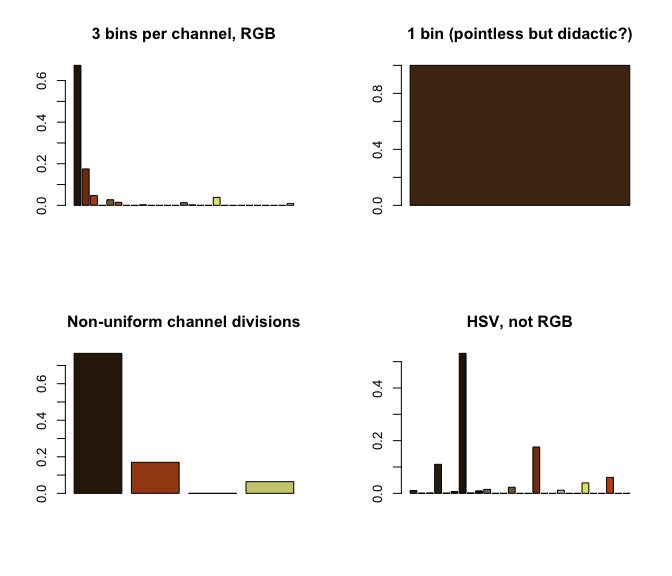In addition to plotting the histogram, the function also returns a dataframe of bin centers and sizes:

defaultHist[1:10, ]
##            r          g          b          Pct
## 1  0.1640884 0.09956622 0.03378178 6.732649e-01
## 2  0.5106194 0.22951992 0.07119379 1.750520e-01
## 3  0.7399364 0.28977472 0.07697233 4.667173e-02
## 4  0.3186791 0.34200206 0.21692466 2.089450e-04
## 5  0.5201913 0.42134362 0.19521215 2.683294e-02
## 6  0.7492967 0.37061505 0.13377436 1.447219e-02
## 7  0.1666667 0.83333333 0.16666667 0.000000e+00
## 8  0.6656863 0.67549020 0.24215686 4.398843e-05
## 9  0.7333498 0.74124238 0.29925853 2.617312e-03
## 10 0.1666667 0.16666667 0.50000000 0.000000e+00

The first three columns of each row are the coordinates in color space of the bin center, and the fourth column is the proportion of pixels in that bin. For example, the first bin, defined by RGB triplet [0.16, 0.10, 0.03], is a very dark brown color, and 6.73e-01 or ~67% of the pixels were assigned to this bin. Two bins with the same set of boundaries may have totally different centers, depending on where the pixels are distributed in that bin. If no pixels are assigned to a bin, its center is defined as the midpoint of the ranges in each channel. So in row 7, the RGB triplet is [0.17, 0.83, 0.17] (a bright green) because the bounds were 0–0.33, 0.67–1, and 0–0.33 and there were no bright green pixels in the image. You can visualize this using plotClusters(), or several cluster sets at once using plotClustersMulti() (see last section).

If you want to get the bin centers and sizes for a set of images at once, use the getHistList() function, which just calls on getImageHist() for all of the images it finds in a folder or set of folders.

images <- dir(system.file("extdata", "Heliconius/", package = "colordistance"), full.names = TRUE)
histList <- colordistance::getHistList(images, bins = 2, plotting = FALSE)
## Warning in colordistance::getHistList(images, bins = 2, plotting = FALSE): RGB and HSV are device-dependent, perceptually non-uniform color spaces. See 'Color spaces' vignette for more information.
## Using 2^3 = 8 total bins
# Output of getHistList() is (you guessed it) a list of dataframes as returned by getImageHist()
histList[]
##           r         g          b          Pct
## 1 0.2496183 0.1779772 0.07175737 0.3149598560
## 2 0.7027810 0.4149608 0.10999870 0.0498903282
## 3 0.4896359 0.5064426 0.41325864 0.0001086372
## 4 0.8776346 0.5702010 0.15412276 0.0940021934
## 5 0.2500000 0.2500000 0.75000000 0.0000000000
## 6 0.5058824 0.4784314 0.50980392 0.0000155196
## 7 0.4941176 0.5450980 0.50588235 0.0000155196
## 8 0.9908927 0.9904837 0.98743203 0.5410286388
# and list elements are named for the image they came from
names(histList)
##  "Heliconius_01" "Heliconius_02" "Heliconius_03" "Heliconius_04"
##  "Heliconius_05" "Heliconius_06" "Heliconius_07" "Heliconius_08"

Unless you have a compelling reason to use k-means instead of the color histogram, I strongly recommend using the histogram binning method.

• Quick. Because we’re just counting how many pixels fall into each of a set of predetermined bounds, computing the histogram takes very little time. K-means (discussed below) takes longer, because it requires iteratively choosing where to place the cluster centers in an image-specific manner.

• Consistent comparisons. Because the bins have the exact same bounds for each image, we can compare the number of pixels in a bin in one image to the number of pixels in the same bin for another image without having to decide if the comparison is a fair one. For example, if you compare color histograms of the French and the Canadian flags, the Canadian flag histogram will have an empty blue bin, but the French flag histogram will have a large blue bin – so we can take into account that one image has a color that is missing from the other image. If you only note the colors that are present, it’s difficult to compare images that have totally different colors.

• Details remain. Even if a color takes up a small part of an image, that detail will still show up in the histogram rather than getting swamped by the more dominant colors.

• Dominant colors aren’t overrepresented. Conversely, if most of an object is a single color, meaning there’s more variation in that color purely because there are more pixels of that color present, they will still all get grouped together in a single bin. K-means, on the other hand, might assign several different clusters to the same color because so many of the pixels fall into that range.

• Artificial distinctions. If you have a cluster of pixels near a boundary, they will be counted separately. The histograms above are a good example: the yellow pixels usually got stuck into two different bins, one with the darker yellow and one with the more pale yellow, even though the yellow pixels in the pixel plot are relatively continuous, because the distribution crossed the blue boundary at 0.5.

• Loss of specific color information. Any binning method will sacrifice color information, but the histogram method treats all pixels within the boundaries of a bin as equivalent. You could have clusters of pixels on opposite sides of a bin which are part of very different color clusters, but which end up treated as a single color which is not actually found on the object because those pixels are averaged out.

For example, say we had an image of a red and yellow checkerboard, so that all the pixels were either [1, 0, 0] (red) or [1, 1, 0] (yellow). If we only divided the blue channel into bins, then all of the red and yellow pixels would end up in the bin where $$B \leq 0.5$$, and their average color would be orange – so our color histogram would indicate that we have a completely orange object, rather than a binary red and yellow one. Since the boundaries are arbitrary, they risk not falling along the “natural” clustering boundaries.

## K-means method

The k-means method is implemented using either getKmeanColors() or getKMeansList(), and dataframes compatible with the analysis functions in colordistance are extracted using extractClusters().

Where the histogram method will always use the same set of bins for an image regardless of its content, k-means uses k-means clustering, which aims to choose a provided number of clusters for a dataset which minimizes the sum of the distances between datapoints and their assigned clusters. So if we had an image of the French flag and used k-means to find 3 clusters, it would return a white cluster, a red cluster, and a blue cluster, each of which contained $$\frac{1}{3}$$ of the pixels in the image. If we wanted to get those same clusters back using the histogram method, we’d have to use a larger number of clusters overall, most of which would be empty – and we might have to manually guess where to put the boundaries for the bins.

The input for the k-means functions are therefore slightly simpler, because rather than specify bins for each channel, the most important variable is just the number of clusters, n:

lower <- rep(0.8, 3)
upper <- rep(1, 3)
kmeans01 <- colordistance::getKMeanColors(Heliconius_08, lower = lower, upper = upper, n = 3)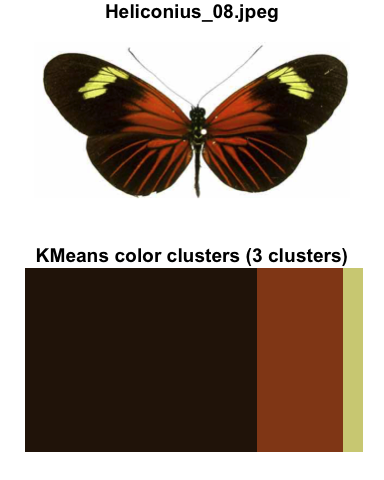Other than the number of clusters, you can also adjust the sample size of pixels on which the fit is performed. Because k-means is iterative and has to perform a fit multiple times for clusters to converge, fitting hundreds of thousands of pixels is computationally expensive. getKmeanColors() gets around this by randomly selecting a number of object pixels equal to sample.size, which is set to a default of 20,000 pixels.

# Using default sample size
system.time(colordistance::getKMeanColors(Heliconius_08, lower = lower, upper = upper, n = 3, plotting = FALSE))
##    user  system elapsed
##   0.073   0.012   0.086
# Using 10,000 instead of 20,000 pixels is slightly faster, but not by much
system.time(colordistance::getKMeanColors(Heliconius_08, lower = lower, upper = upper, n = 3, plotting = FALSE, sample.size = 10000))
##    user  system elapsed
##   0.049   0.013   0.062
# Using all pixels instead of sample takes 5x longer - and this is a very low-res image!
system.time(colordistance::getKMeanColors(Heliconius_08, lower = lower, upper = upper, n = 3, plotting = FALSE, sample.size = FALSE))
## Performing fit on all pixels (slow for large images).
##    user  system elapsed
##   0.270   0.035   0.305

Unlike the histogram method, k-means will not return the exact same clusters every time you run it, even if you perform the fit on the whole image rather than a subset of pixels – this is also a feature of the iterative behavior. You can minimize the differences by increasing the values of iter.max and nstart, which are passed to the kmeans() function of the stats package. (As you might guess, this makes the function slower.) Unless the image has extremely high color complexity, however, the differences should be minor and in my experience don’t affect analyses.

The output of getKMeanColors() is a kmeans() fit object, a list which contains the cluster centers, a vector indicating which cluster each pixel has been assigned, and a series of statistical measures for the goodness of the k-means fit. This more complete information might be useful for other analyses, but for the rest of the colordistance functions, you’ll then want to run extractClusters() to get a dataframe like the one returned by getImageHist():

kmeansDF <- colordistance::extractClusters(kmeans01)
print(kmeansDF)
##           R          G          B     Pct
## 1 0.8177902 0.81147841 0.51645860 0.05890
## 2 0.5753375 0.28141523 0.10104392 0.25385
## 3 0.1688634 0.09925977 0.03233204 0.68725

Looks pretty similar to the one returned by getImageHist() above, with the obvious difference that there are only 3 clusters, and none of them are empty. To run the analysis for all of the images in a set, use getKmeansList() followed by extractClusters():

# In order to see the clusters for each image, set plotting to TRUE and optionally pausing to TRUE as well
kmeans02 <- colordistance::getKMeansList(images, bins = 3, lower = lower, upper = upper, plotting = F)
kmeansClusters <- colordistance::extractClusters(kmeans02, ordering = T)
head(kmeansClusters, 3)
## $Heliconius_01 ## R G B Pct ## 1 0.2254525 0.1565498 0.05518518 0.5677 ## 2 0.8495645 0.5508927 0.16717650 0.2796 ## 3 0.4327825 0.3101981 0.14859329 0.1527 ## ##$Heliconius_02
##           R         G          B     Pct
## 1 0.2727082 0.1920413 0.07270281 0.32575
## 3 0.8386857 0.5268091 0.15168034 0.47945
## 2 0.5648559 0.3596167 0.11749607 0.19480
##
## \$Heliconius_03
##           R         G          B     Pct
## 3 0.2987156 0.2131830 0.07187796 0.32930
## 1 0.8175992 0.5481564 0.14548703 0.56355
## 2 0.9275251 0.8881063 0.44295975 0.10715

The result is nearly identical in structure to the result of getHistList(), but note the ordering on the far left. Because ordering=T in extractClusters(), the clusters were reordered so that the most similar clusters across each cluster set were in the same row. So the black/dark brown cluster is in the same row for every dataset, and so on. This is important for later analyses in order to compare equivalent colors to each other rather than comparing the red on one butterfly to the black of another, and so on.

• Image-specific color choices. If an image has important color variation in a narrow region of color space, k-means may be able to pick up on it more easily than a color histogram would. If an image has lots of blue and blue-green, for example, then you might need to use a large number of bins for a color histogram in order to draw a boundary between these two colors, and you might end up dividing continuous color clusters in the process. With k-means, since the two colors form natural clusters, they will be separated if the appropriate number of clusters is provided.

• Color palette extraction. A popular use of k-means clustering with images is to extract a color palette to examine and isolate the dominant colors of an image. If you care more about getting the main colors out than about comparing that image to other images for color similarity, this method is more useful than a histogram.

• Objects with the same set of dominant colors. If your dataset is mostly objects of images with the same approximate colors, using a histogram might equal out these more subtle differences and you’d end up with near-identical histograms because different colors fall into the same bin. But note that setting bin.avg=T for histogram functions does retain information on where in each bin the pixels center, so bins aren’t totally equalized.

• Misses details. If a very small portion of an image is a different color, this detail will tend to get swallowed up by a cluster unless a very large number of clusters is specified. This is because k-means clustering tries to minimize the sum of the distances between pixels and their clusters. If a detail color is a small fraction of the pixels, then they don’t contribute much to the sum, and that number can be more effectively decreased by adding more clusters in pixel-dense regions of color space, leaving details out of the cluster set.

• Divides dominant colors into near-identical clusters. The flip side of leaving out details is that dominant colors are over-represented. When a color takes up a large portion of an image, it tends to get broken up into several clusters, because the greatest decrease in pixel-cluster distance can be achieved by adding another cluster in a pixel-dense region. 3 clusters worked well for the butterfly picture earlier, but when we use the default of n=10, we get several black/dark brown clusters, several orange/red ones, and several yellow ones, even though it doesn’t make intuitive sense to divide these colors up this way.

colordistance::getKMeanColors(Heliconius_08, lower = lower, upper = upper, return.clust = FALSE)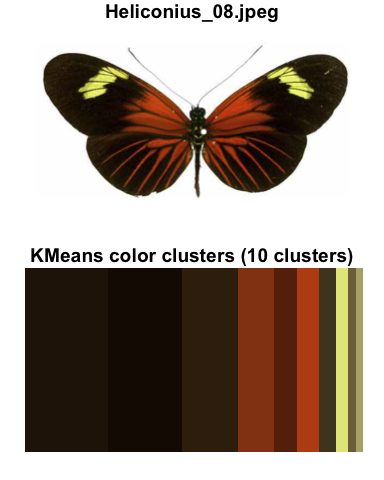• Comparisons for dissimilar objects difficult. If we have two black and white objects, we know it makes sense to compare the amount of black in one to the amount of black in the other and same for the white, rather than the black in one to the white in the other. But what if you’re comparing a black and white object to a blue and yellow one? Or an all-black object to one with 10 distinct colors? Unlike the histogram method, which uses empty bins to register the lack of a color in an image, k-means only returns clusters which are present in an image.

• Requires same number of clusters for every image. If images in a dataset have a wide range of color complexity, it can be difficult to choose the right number of clusters – too many, and simpler images will have their colors divided up into extremely similar but still separate clusters; too few, and details in more complex images will be obscured.

• Slow. k-means clustering is iterative and usually working with thousands of data points to perform a fit, while color histograms just have to count how many pixels fall into certain regions, no fit required. K-means binning usually takes much longer than color histograms, which can make a big difference if you’re working with a large set of images or high-resolution images.

## Choosing a binning method & parameters

The best binning method will depend on the dataset and the details being emphasized for analysis. The pros and cons listed above should help clarify what the effects of each method are likely to be, but there’s no harm in trying out a few different methods. I recommend starting out with the color histogram method unless you have a good reason to use k-means instead – the clusters may not look as intuitive, but the comparisons between images tend to have more statistical merit. Even if a color gets broken up across two bins, it will usually get broken up the same way in two different images, so the histograms will still look similar. And when a color is absent, that’s noted via an empty bin so we can compare presence to absence across images. That said, if your primary concern is extracting dominant colors in an image rather than making meaningful comparisons, k-means might be the way to go. If one were superior to the other in all respects, they wouldn’t both be included!

The nice thing about color clustering is that, unlike most statistical analyses, it’s trying to quantify something which is for the most part fairly visually intuitive – it should be obvious if the parameters you’re choosing are returning scores that don’t make a lot of sense, since we’re ranking images by color similarity. If you try k-means and it fails to cluster visually similar objects together, then k-means probably isn’t the right choice; if you choose too few bins and dissimilar objects are scoring as similar, you probably need to use more bins, and so on.

imageClusterPipeline() is a single function that goes from raw images to distance matrix in one line, making it easy to tweak parameters and methods. Setting clusterMethod="hist" or clusterMethod="kmeans" will toggle between the two methods.

## Cheatsheet

Histogram method

par(mfrow=c(1, 3))
# Get and plot histogram for a single image
hist01 <- colordistance::getImageHist(Heliconius_08, bins = 2, plotting = TRUE, title = "RGB, 2 bins per channel", lower = lower, upper = upper)
## RGB and HSV are device-dependent, perceptually non-uniform color spaces. See 'Color spaces' vignette for more information.
##
## Using 2^3 = 8 total bins
# Use the bin center as the cluster value instead of the average pixel location (note the difference between this and when bin.avg=F)
hist02 <- colordistance::getImageHist(Heliconius_08, bins = 2, plotting = TRUE, title = "bin.avg = F", lower = lower, upper = upper, bin.avg = FALSE)
## RGB and HSV are device-dependent, perceptually non-uniform color spaces. See 'Color spaces' vignette for more information.
##
##
## Using 2^3 = 8 total bins
# Use different number of bins for each channel; use HSV instead of RGB
hist03 <- colordistance::getImageHist(Heliconius_08, bins=c(8, 1, 2), plotting=TRUE, hsv=TRUE, title="HSV, 8 hue, 1 sat, 2 val", lower=lower, upper=upper)
## RGB and HSV are device-dependent, perceptually non-uniform color spaces. See 'Color spaces' vignette for more information.
##
## Using 8*1*2 = 16 bins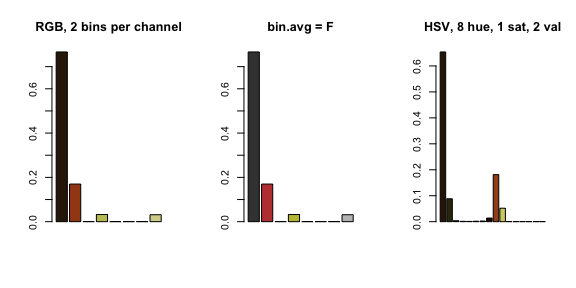# Get histograms for a set of images
histMulti <- colordistance::getHistList(images, bins=2, plotting=FALSE, lower=lower, upper=upper)

K-means method

lower <- rep(0.8, 3)
upper <- rep(1, 3)

# Use defaults
kmeans01 <- colordistance::getKMeanColors(Heliconius_08, n=3, plotting=FALSE, lower=lower, upper=upper)
kmeansDF <- colordistance::extractClusters(kmeans01)

# Use a larger sample size
kmeans02 <- colordistance::getKMeanColors(Heliconius_08, n=3, plotting=FALSE, sample.size = 30000, lower=lower, upper=upper)
kmeansDF2 <- colordistance::extractClusters(kmeans02)

# Don't return clusters as a dataframe
colordistance::getKMeanColors(Heliconius_08, n=15, plotting=FALSE, return.clustlust=FALSE, lower=lower, upper=upper)
# For whole dataset
kmeans03 <- colordistance::getKMeansList(images, n=3, plotting=FALSE, lower=lower, upper=upper)
kmeansList <- colordistance::extractClusters(kmeans03)

Quick comparison

# If we use the same number of clusters for both the histogram and k-means methods, how different do the clusters look?
# Not run in this vignette, but produces 3D, interactive plots!
histExample <- colordistance::getHistList(images, lower = lower, upper = upper)

kmeansExample <- colordistance::extractClusters(colordistance::getKMeansList(images, bins = 27, lower = lower, upper = upper))

colordistance::plotClustersMulti(histExample, title = "Histogram method")

colordistance::plotClustersMulti(kmeansExample, title = "K-means method")



Meyer, Axel. 2006. “Repeating Patterns of Mimicry.” PLOS Biology 4 (10). Public Library of Science: 1–3. https://doi.org/10.1371/journal.pbio.0040341.

1. Although the 0–255 intensity scale is common for RGB images, R reads images in on a 0–1 intensity scale; unless otherwise stated, the 0–1 scale should be assumed for any colordistance` documentation and examples.